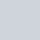Latest MCQs
Home > MCQs > Math > A man traveling an hour by car and another hour by plane covers a total distance of 850 Kms. If the average speed of plane taken by the man was 16 times that of the car. What was the distance covered by the car.

# A man traveling an hour by car and another hour by plane covers a total distance of 850 Kms. If the average speed of plane taken by the man was 16 times that of the car. What was the distance covered by the car.

A.40
B.50
C.60
D.65